## Linear Combination

A set of vectors of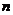dimensions can be represented as a linear combination ofother vectors. A linear combination of vectors involves both addition and scalar multiplication of vectors.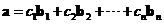## Span

Geometrically, a set of all linear combination of vectors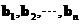generates or spans a space. Any point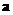in that space can be represented as a linear combination of vectorsprovided that these vectors cannot be represented as a linear combination of one another. In other words, vectorsspandimensional space with a minimum number of vectorssuch that the only necessary vectors to be included.

## Basis Vector

The vectorsare called basis vectors because they are the basis of a space. The space (or multidimensional space) is indicated by the coordinate system . The scalars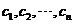are called the coordinate because they represent the coordinate of the space. Formally, we say that a basis is a set of linearly independent vectors which span the space.

For example
In 2-dimensional Euclidean space (that is the space in an ordinary coordinate system that you have learned since grade school), you represent the coordinate of a point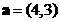as a linear combination of the standard unit vectors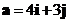.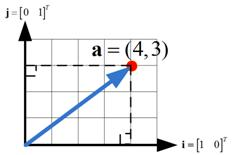You can represent the same coordinate of a pointas a linear combination of other vectors such as vectors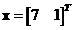and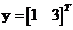to form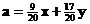(see Change of Basis to see how we get the coordinate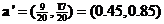in the new coordinate system). That is to say that the coordinate of pointin Euclidean coordinate system is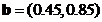in the new coordinate system. Since the vectorandform a coordinate system, we call them basis vectors. Notice that the coordinate is the scalar multiple to the length of the basis vector. Coordinate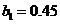is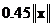and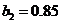is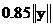in the direction of respective basis vectors as shown in the figure below. Notice that the coordinate system does not need to be orthogonal (i.e. perpendicular to each other). Geometrically, the coordinate of a point in the new coordinate system is drawn by taking a parallel line to the basis vectors to cross the other basis vectors.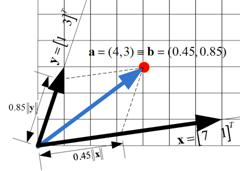Now let us take a counter example. The same point that we represent in Euclidean coordinate system ascannot be represented as linear combination of vectorand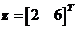. Algebraically, no scalar coordinate can be set to create the linear combination. Thus, the two vectors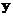and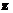cannot form a coordinate system. This is because the two vectors are collinear (lie on the same line or parallel lines) as you can see in the figure below. Geometrically, by taking a parallel line to one of the vector you cannot find a crossing point to the other vector.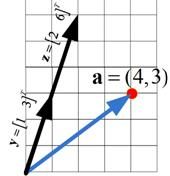To form basis vectors, we horizontally concatenate the vectors of the same dimension into a matrix, and then reduce the matrix into matrix RREF . The corresponding columns that contains the leading 1s in the matrix RREF is the basis vectors.

## Note:

Suppose we are using vectors ofdimensions (i.e. the vector haselements) and we have a set of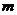vectors (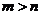), the set of vector spandimensional space but any of the vector can be expressed as a linear combination of the othervectors. Thus, the set of vectors is not a basis. By removing the appropriate vectors up tovectors, we can reduce the set into basis vectors.

Similarly, if we are using vectors ofdimensions (i.e. the vector haselements) and we have a set oflinearly independent vectors (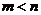), then the set of vector does not spandimensional space. Thus, the set of vectors is not a basis. By adding the appropriate linearly independent vectors up tovectors, we can make the set into basis vectors.

In general, there are two conditions for a set of vectors to form basis vectors:

1. The basis set must have as many basis vectors as the number of dimensions (we say that the basis vector must span the space). If the vector length isdimensions, then we will havebasis vectors.
2. No basis vector can be put as a sum of the other basis vectors (we say that the basis vectors must be linearly independent).

In short, a set of vectors that can form a coordinate system is called basis vectors . Basis vectors are equivalent to linearly independent vectors (as long as we keep the number of basis vectors equal to its dimensions).

In the next topic , you will learn how to test if a set of vectors is linearly independent or linearly dependent vectors.